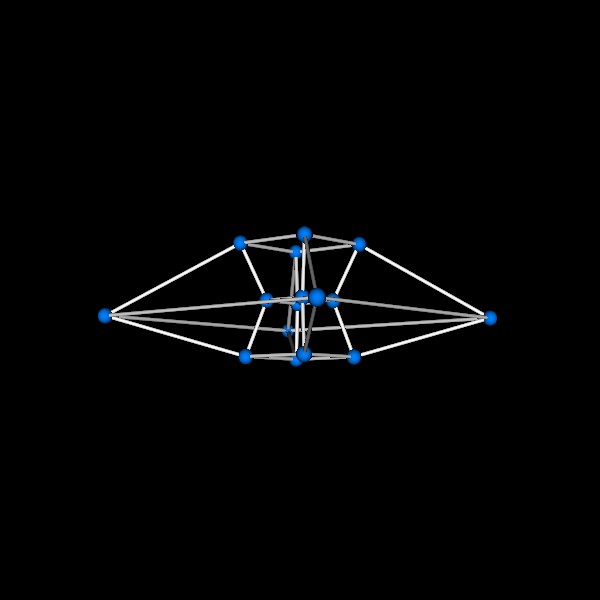# Tesseract (Hypercube)¶

A tesseract is a four-dimensional cube. A tesseract can be unfolded into eight cubes, Just as a cube can be unfolded into eight squares.

First, import some useful functions

```import numpy as np
from fury import utils, actor, window
from fury.ui import TextBlock2D
import itertools
```

Let’s define some variables and their descriptions:

Use wireframe = True to show wireframe like representation of the tesseract wireframe = False will render it with point actor on each vertex.

```wireframe = False

# p_color: color of the point actor (default: (0, 0.5, 1, 1))
# e_color: color of the line actor (default: (1, 1, 1, 1))
# dtheta: change in `angle` on each iteration. It determines the "speed" of the
#        animation. Increase dtheta to increase speed of rotation, It may
#        result in less smoother rotation (default: 0.02)
# angle: defines the angle to be rotated to perform the animation, It changes
#        as we run the `callback` method later on. (initial value: 0)

p_color = np.array([0, 0.5, 1, 1])
e_color = np.array([1, 1, 1, 1])
dtheta = 0.02
angle = 0
```

Let’s define vertices for our 4D cube, verts4D contains the coordinates of our 4D tesseract.

```verts3D = np.array(
[[1, 1, 1],
[1, -1, 1],
[-1, -1, 1],
[-1, 1, 1],
[-1, 1, -1],
[1, 1, -1],
[1, -1, -1],
[-1, -1, -1]]
)

# We can use primitive.box alternatively to get the cube's 3-D vertices.

u = np.insert(verts3D, 3, 1, axis=1)
v = np.insert(verts3D, 3, -1, axis=1)
verts4D = np.append(u, v, axis=0)
```

We define a rotate4D function that takes 4D matrix as parameter and rotates it in XY plane (Z axis) and ZW plane (an imaginary axis), projects it to the 3D plane so that we can render it in a scene.

```def rotate4D(verts4D):
cos = np.cos(angle)
sin = np.sin(angle)
rotation4d_xy = np.array(
[[cos, -sin, 0, 0],
[sin, cos, 0, 0],
[0, 0, 1, 0],
[0, 0, 0, 1]])
rotation4d_zw = np.array(
[[1, 0, 0, 0],
[0, 1, 0, 0],
[0, 0, cos, -sin],
[0, 0, sin, cos]])
distance = 2
projected_marix = np.zeros((16, 3))
for i, vert in enumerate(verts4D):
rotated_3D = np.dot(rotation4d_xy, vert)
rotated_3D = np.dot(rotation4d_zw, rotated_3D)
w = 1 / (distance - rotated_3D)
proj_mat4D = np.array(
[[w, 0, 0, 0],
[0, w, 0, 0],
[0, 0, w, 0]]
)

projeced_mat3D = np.dot(proj_mat4D, rotated_3D)
projected_marix[i] = projeced_mat3D  # vertices to be proj (3D)
return projected_marix
```

Now, We have 4D points projected to 3D. Let’s define a function to connect lines.

```def connect_points(verts3D):
lines = np.array([])
len_vert = len(verts3D)

for i in range(len_vert-1):
if i < 8:
lines = np.append(lines, [verts3D[i], verts3D[i+8]])
if i == 7:
pass
else:
lines = np.append(lines, [verts3D[i], verts3D[i+1]])
if i % 4 == 0:
lines = np.append(lines, [verts3D[i], verts3D[i+3]])

for i in range(3):
lines = np.append(lines, [verts3D[i], verts3D[i+5]])
lines = np.append(lines, [verts3D[i+8], verts3D[i+5+8]])

return np.reshape(lines, (-1, 2, 3))
```

Creating a scene object and configuring the camera’s position

```scene = window.Scene()
scene.set_camera(position=(0, 10, -1), focal_point=(0.0, 0.0, 0.0),
view_up=(0.0, 0.0, 0.0))
showm = window.ShowManager(scene,
size=(1920, 1080), order_transparent=True)
showm.initialize()
```

Creating vertices and points actors

```verts3D = rotate4D(verts4D)
if not wireframe:
points = actor.point(verts3D, colors=p_color)
point_verts = utils.vertices_from_actor(points)
no_vertices = len(point_verts) / 16
initial_verts = point_verts.copy() - \
np.repeat(verts3D, no_vertices, axis=0)

```

Connecting points with lines actor

```lines = connect_points(verts3D)
edges = actor.line(lines=lines, colors=e_color,
lod=False, fake_tube=True, linewidth=4)
lines_verts = utils.vertices_from_actor(edges)
initial_lines = lines_verts.copy() - np.reshape(lines, (-1, 3))

```

Initializing text box to display the name

```tb = TextBlock2D(text="Tesseract", position=(900, 950),
font_size=20)
```

Define a timer_callback in which we’ll update the vertices of point and lines actor using rotate4D.

```counter = itertools.count()
end = 200

def timer_callback(_obj, _event):
global verts3D, angle
cnt = next(counter)
verts3D = rotate4D(verts4D)
if not wireframe:
point_verts[:] = initial_verts + \
np.repeat(verts3D, no_vertices, axis=0)
utils.update_actor(points)

lines = connect_points(verts3D)
lines_verts[:] = initial_lines + \
np.reshape(lines, (-1, 3))
utils.update_actor(edges)

showm.render()
angle += dtheta

if cnt == end:
showm.exit()
```

Run every 20 milliseconds

```showm.add_timer_callback(True, 20, timer_callback)
showm.start()
window.record(showm.scene, size=(600, 600), out_path="viz_tesseract.png")
```Total running time of the script: ( 0 minutes 5.180 seconds)

Gallery generated by Sphinx-Gallery